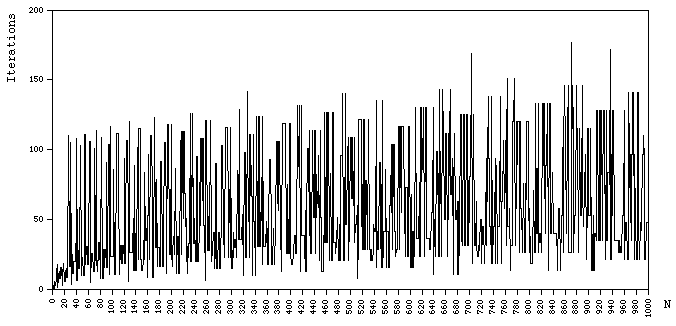# Decision procedure for "oneness"

Written by Paul Bourke
December 1992

Listen (mov or midi) to the oneness for the first 300 integers

A surprising source of chaos in number theory arises from the so called "oneness" of any positive number. Consider iteratively performing the following procedure starting with any positive number, if the number is even then divide it by 2, if odd then multiply it by 3 and add 1. The number of steps before this series reaches 1 will be called the oneness of the original number. For example the oneness of 3 is 7, the sequence is 3, 10, 5, 16, 8, 4, 2, 1.

A graph showing the oneness of the first 1000 integers is given belowNote that two neighbouring numbers can have vastly different oneness values. for example, the oneness of 47 is 104 but the oneness of 48 in only 11. The oneness of 737 is 139 while the oneness of 738 is 20.

It turns out that there is no decision procedure to determine what the oneness is! The only way to find out is to run the sequence which may be infinite. (?)